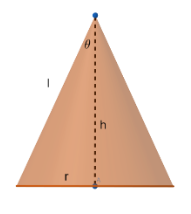Courses
Courses for Kids
Free study material
Free LIVE classes
More# Show that semi-vertical angle of right circular cone of given surface area and maximum volume a given slant height $l$ is ${{\tan }^{-1}}\left( \dfrac{1}{2\sqrt{2}} \right)$.Verified
326.7k+ views
Hint: First find the surface area of the cone. After that, take $l=\sqrt{{{h}^{2}}+{{r}^{2}}}$ and compare to get the volume. Then for maximum volume, $\dfrac{dV}{dr}=0$, from this you will get the value of $S$ and substitute in $l$, you will get the value of $l$ in terms of $r$ and also you will get the value of $h$. The take $\tan$ of semi vertical angle and you will get the answer.Now the surface area of the cone will be $S=\pi {{r}^{2}}+\pi rl$ where r is the radius and l is the slant height of the cone.
Now $l=\dfrac{S-\pi {{r}^{2}}}{\pi r}$……….. (1)
Also from above figure we know that ${{h}^{2}}+{{r}^{2}}={{l}^{2}}$, where $h$ is the vertical height of the cone.
So we get, $l=\sqrt{{{h}^{2}}+{{r}^{2}}}$ ……….(2)
From (1) and (2), we get,
$\sqrt{{{h}^{2}}+{{r}^{2}}}=\dfrac{S-\pi {{r}^{2}}}{\pi r}$
Squaring both sides we get,
\begin{align} & {{h}^{2}}+{{r}^{2}}={{\left( \dfrac{S-\pi {{r}^{2}}}{\pi r} \right)}^{2}} \\ & {{h}^{2}}=\left( \dfrac{{{S}^{2}}+{{\pi }^{2}}{{r}^{4}}-2\pi S{{r}^{2}}}{{{\pi }^{2}}{{r}^{2}}} \right)-{{r}^{2}} \\ & {{h}^{2}}=\left( \dfrac{{{S}^{2}}+{{\pi }^{2}}{{r}^{4}}-2\pi S{{r}^{2}}-{{\pi }^{2}}{{r}^{4}}}{{{\pi }^{2}}{{r}^{2}}} \right) \\ & {{h}^{2}}=\left( \dfrac{{{S}^{2}}-2\pi S{{r}^{2}}}{{{\pi }^{2}}{{r}^{2}}} \right) \\ & h=\dfrac{\sqrt{{{S}^{2}}-2\pi S{{r}^{2}}}}{\pi r} \\ \end{align}
Now volume of cone V$=\dfrac{1}{3}\pi {{r}^{2}}h=\dfrac{1}{3}\pi {{r}^{2}}\dfrac{\sqrt{{{S}^{2}}-2\pi S{{r}^{2}}}}{\pi r}$
V$=\dfrac{r}{3}\sqrt{{{S}^{2}}-2\pi S{{r}^{2}}}$
For maximum volume, $\dfrac{dV}{dr}=0$.
\begin{align} & \dfrac{1}{3}\sqrt{{{S}^{2}}-2\pi S{{r}^{2}}}+\dfrac{r}{3}\dfrac{-4\pi Sr}{2\sqrt{{{S}^{2}}-2\pi S{{r}^{2}}}}=0 \\ & \dfrac{1}{3}\sqrt{{{S}^{2}}-2\pi S{{r}^{2}}}=\dfrac{r}{3}\dfrac{2\pi Sr}{\sqrt{{{S}^{2}}-2\pi S{{r}^{2}}}} \\ & {{S}^{2}}=4\pi S{{r}^{2}} \\ \end{align}
we know that, $S\ne 0$.
So $S=4\pi {{r}^{2}}$
Now taking (1) and substituting the $S=4\pi {{r}^{2}}$ we get,
$l=\dfrac{4\pi {{r}^{2}}-\pi {{r}^{2}}}{\pi r}=3r$
So $l=\sqrt{{{h}^{2}}+{{r}^{2}}}$
\begin{align} & 9{{r}^{2}}={{h}^{2}}+{{r}^{2}} \\ & h=2\sqrt{2}r \\ \end{align}
Now, let $\theta$ be the semi-vertical angle of the cone.
\begin{align} & \tan \theta =\dfrac{r}{h}=\dfrac{r}{2\sqrt{2}r} \\ & \tan \theta =\dfrac{1}{2\sqrt{2}} \\ \end{align}
So $\theta ={{\tan }^{-1}}\left( \dfrac{1}{2\sqrt{2}} \right)$
Hence proved.

Note: This question deals with maxima hence differentiation is involved. Few relations like the one between r, l & h are determined based on the geometry of the figure. For finding the condition for maximum volume, volume is differentiated wrt the radius and equated to 0. Avoid mistakes while differentiating.
Last updated date: 02nd Jun 2023
Total views: 326.7k
Views today: 6.83k